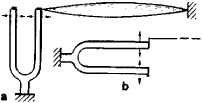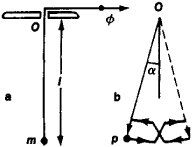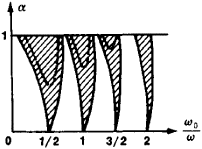# Parametric Excitation of Oscillations

## Parametric Excitation of Oscillations

the excitation of oscillations in an oscillatory system through the periodic variation of the value of one of the system’s oscillation parameters—that is, the parameters on whose values the system’s potential energy, kinetic energy, and period of natural oscillations essentially depend. Oscillations can be excited parametrically in any oscillatory system, whether mechanical or electric. An example is an oscillatory circuit formed by a capacitor and an induction coil when the capacitance of the capacitor or the inductance of the coil is varied periodically.

Parametric excitation of oscillations occurs when the ratio w0/w is close to n/2, where w0 is the angular frequency of one of the system’s natural oscillations, w) is the angular frequency of the parameter variations, and n = 1, 2, 3 …. Oscillations can then be excited in the system at a frequency that is close to w0 and is exactly equal to w /2, or w, or 3w/2, and so on. Parametric excitation of oscillations occurs most easily and the excited oscillations are most intense when w0/w) ≈ ½.Figure 1. (a) Parametric excitation of vibrations of a string, (b) forced vibration of a string

A string that is attached at one end to a prong of a tuning fork provides a classic example of parametric excitation: by periodically varying the string’s tension, intense transverse oscillations are induced in the string (Figure 1,a). The parametric excitation is easiest when the period of one of the string’s natural vibrations (the string’s fundamental or one of its harmonics) is approximately twice the period of the tuning fork’s vibration. For the usual excitation of a forced vibration of a string (Figure 1,b), where the period is equal to the period of the tuning fork’s vibration, resonance occurs whenever the period of the tuning fork’s vibration coincides with the period of one of the natural vibrations of the string. Thus the phenomenon of parametric excitation of oscillations is in this respect like resonance in the case of the usual excitation of forced vibrations. For this reason the phenomenon is often called parametric resonance.Figure 2. (a) Pendulum mechanism with a suspension of variable length, (b) schematic of the motion of the pendulum body in one period

The nature of the phenomenon can be illustrated by a pendulum of a mass m suspended on a thread whose length l can be varied (Figure 2,a). The oscillation period of the pendulum depends on the length of the suspension. Thus, by varying the length with a period that is, for example, half the period of the pendulum’s natural oscillation, this oscillation can be excited parametrically. After imparting a small natural oscillation to the pendulum, we lengthen the thread each time the pendulum is at one of the outermost positions and shorten the thread when the pendulum passes through the middle position in either direction (Figure 2,b). The tension in the thread not only counterbalances the component of the force of gravity in the direction of the thread mg cos a (where a is the angle of deflection of the pendulum from the vertical) but also imparts a centripetal acceleration v2/l to the body. Thus, the tension in the thread is F = mg cos α + mv2/2; that is, F has its smallest value when the pendulum is at the outermost positions, where v = 0 and α ≠ 0. When the thread is shortened in the middle position, the external force Φ performs more work than the negative work that is performed when the thread is lengthened at the outermost positions. As a result, the external force performs positive work during each period of the oscillation. If this work exceeds the energy losses of the oscillation in the system during the period, the energy, and hence the amplitude, of the pendulum’s oscillation will increase. Consequently, the initial natural oscillation imparted to the pendulum can have an arbitrarily small amplitude. In particular, there could be fluctuational oscillations that are inevitably set up in oscillatory systems by various random factors and that have a continuous spectrum with all the possible phases in their harmonic components. Thus, regardless of the phase of the periodic variations in the length of the suspension, the pendulum will always have a small natural oscillation for which these variations occur in the required phase; as a result, the amplitude of this particular natural vibration will increase.

During parametric excitation of oscillations the equilibrium state becomes unstable owing to the periodic action on a parameter, and the system starts to build up oscillations around the equilibrium position. The oscillations, however, do not increase without limit because when the amplitude and velocities of the oscillations attain larger values, the oscillatory system begins to act as a nonlinear system and the growth of the oscillations is halted.Figure 3. Regions in which parametric excitation of oscillations is possible

As has already been shown, the regions where the equilibrium state is unstable and parametric excitation of oscillations occurs lie close to the values w0/w) = 1/2, 1, 3/2 … (Figure 3) and depend on the relative amplitude of the variations of the parameter a. The greater this amplitude, the broader the region—that is, the greater the difference between w0/w and 1/2, 1, and so on at which parametric excitation of oscillations is observed. Outside of the regions of instability, parametric excitation of oscillations does not occur and there are no oscillations in the system. In contrast, for “ordinary” excitation of forced vibrations, weak forced oscillations occur even a long way from resonance. Near the values w0/w) = 1/2, 1, 3/2, … oscillations are parametrically excited, as is evident from Figure 3, by arbitrarily small amplitudes of the parameter variations. This situation is explainable by our neglect of the energy losses that always exist in a real oscillatory system. If the energy losses are taken into account, the regions where the equilibrium state is unstable (the broken lines in Figure 3) become smaller. As might be expected, where losses are present, instability, even in the absence of mistuning, occurs only when the amplitude of the parameter variations is sufficiently high— that is, when the energy contribution from the periodic variation of the parameter exceeds the losses. Thus, as a result of the energy losses there is always a threshold for the parametric excitation of oscillations. In systems having large losses this threshold rises above the limit of possible parameter variations —first for the higher values of w0/w and then for w0/w = 1/2; that is, the phenomenon of parametric excitation of oscillations cannot occur at all.

### REFERENCES

Gorelik, G. S. Kolebaniia i volny, 2nd ed. Moscow, 1959. Chapter 3, subsec. 9.
Mandel’shtam, L. I. Poln. sobr. trudov, vol. 4. Moscow, 1955. (Lektsii po kolebaniiam, part 1, lectures 18–19.)

S. E. KHAIKIN

Site: Follow: Share:
Open / Close